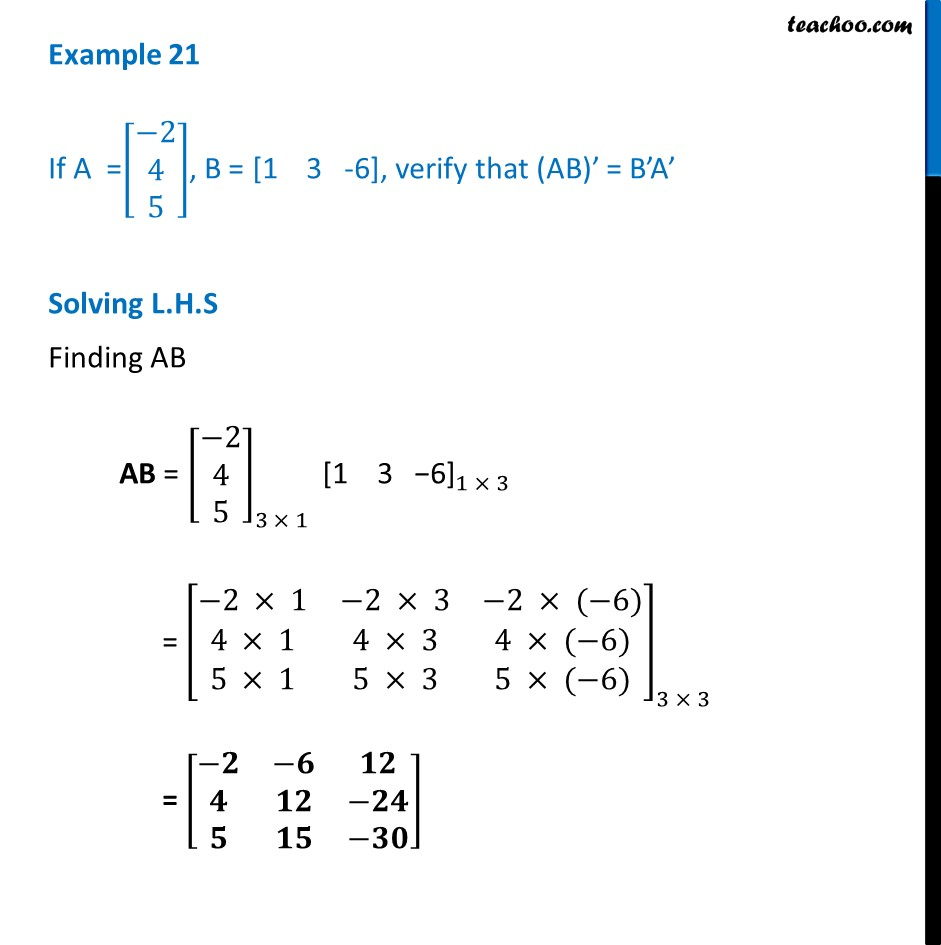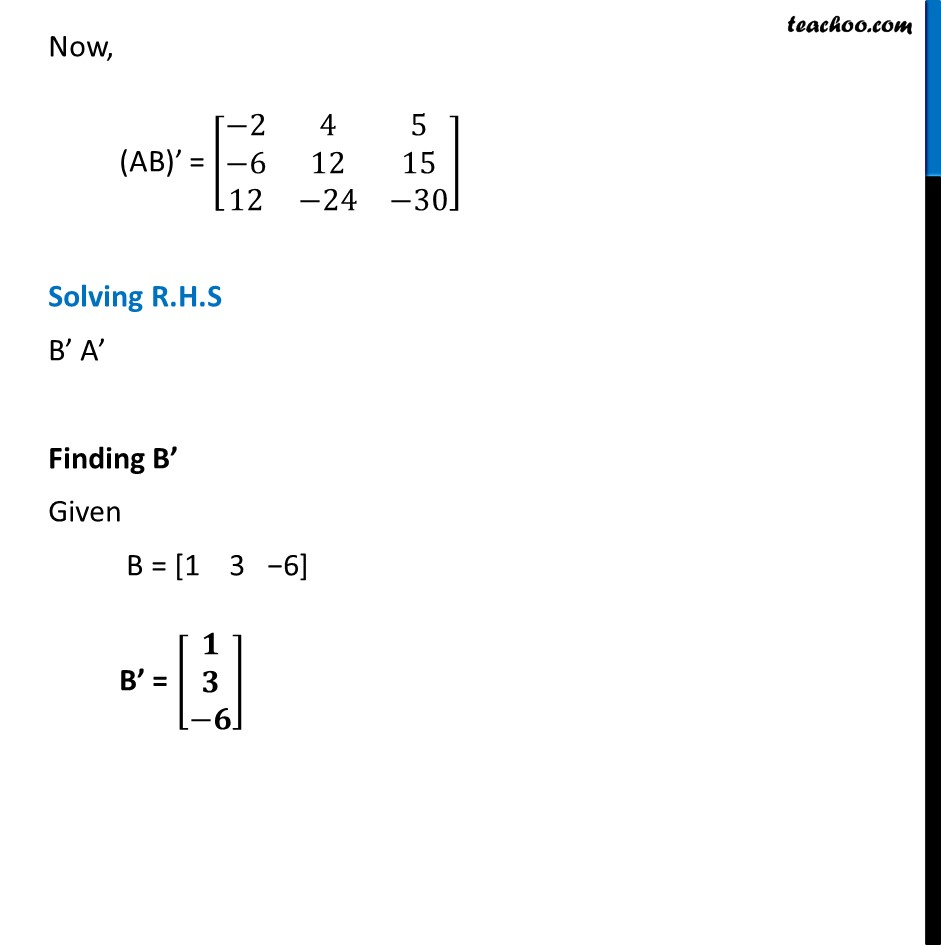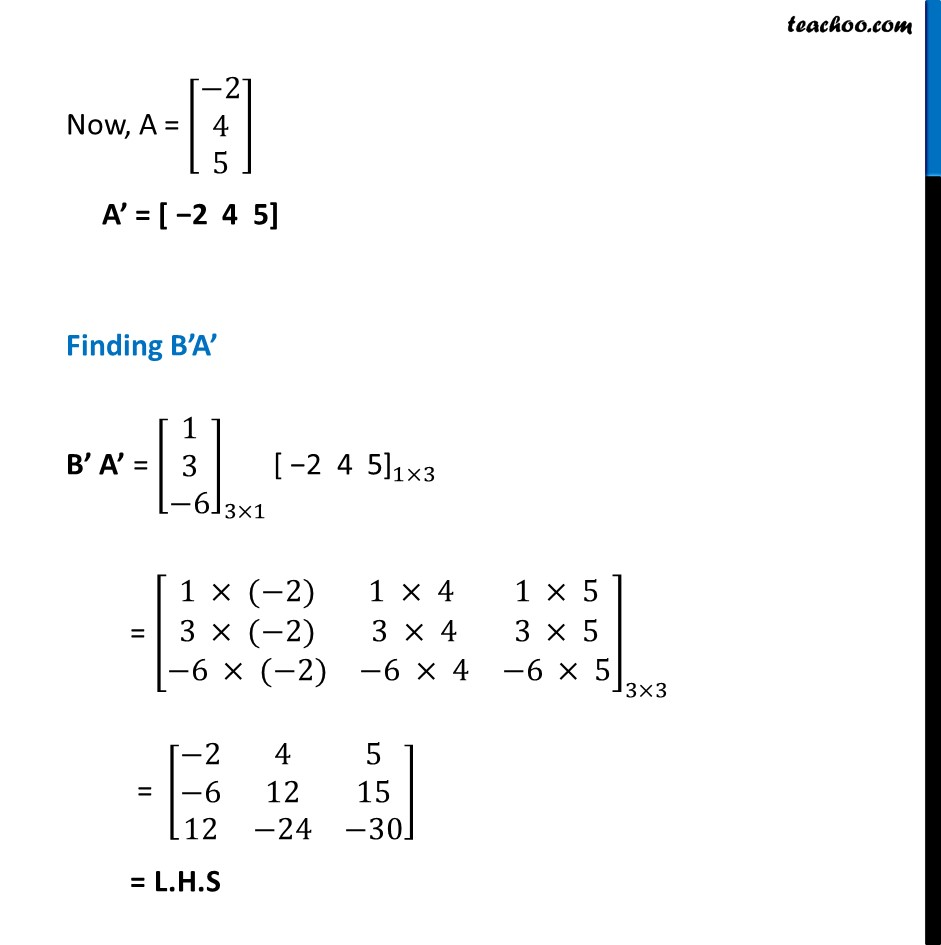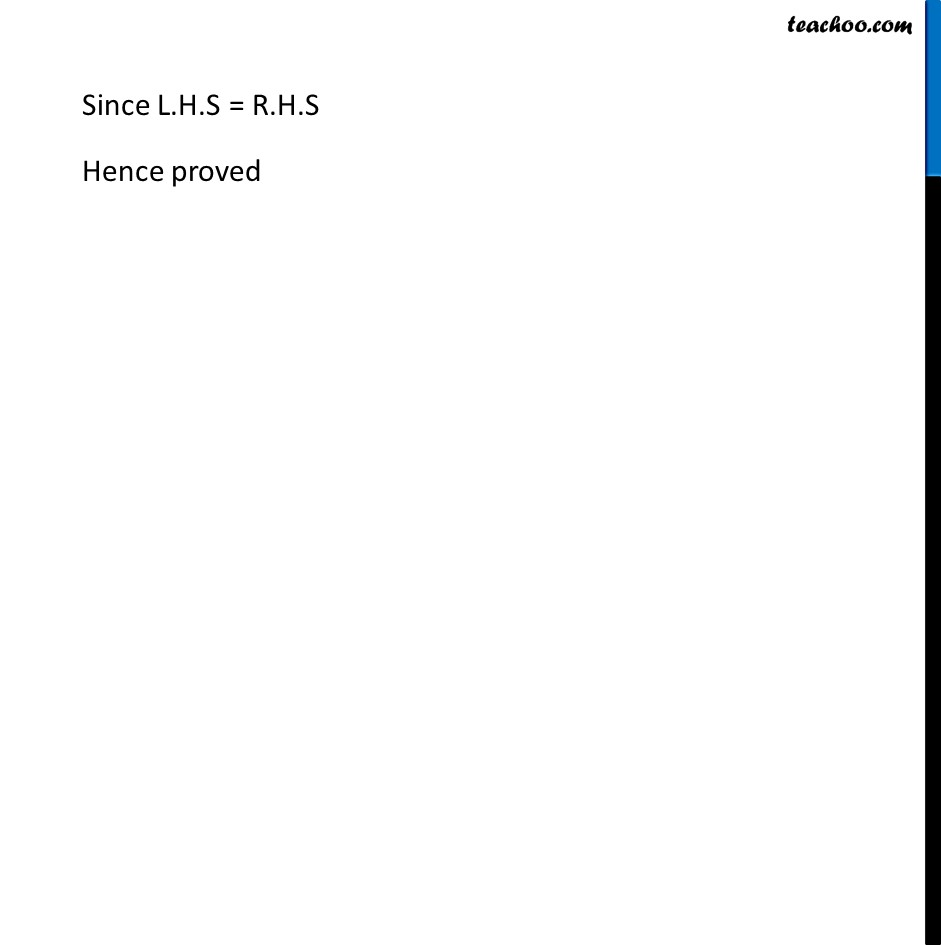Examples

Chapter 3 Class 12 Matrices
Serial order wiseLearn in your speed, with individual attention - Teachoo Maths 1-on-1 Class

### Transcript

Example 21 If A =[■8(−[email protected]@5)], B = [1 3 -6], verify that (AB)’ = B’A’ Solving L.H.S Finding AB AB = [■8(−[email protected]@5)]_(3 × 1) 〖"[1 3 −6]" 〗_(1 × 3) = [■8(−2 × 1&−2 × 3&−2 × (−6)@4 × 1&4 × 3&4 × (−6)@5 × 1&5 × 3&5 × (−6))]_(3 × 3) = [■8(−𝟐&−𝟔&𝟏𝟐@𝟒&𝟏𝟐&−𝟐𝟒@𝟓&𝟏𝟓&−𝟑𝟎)] Now, (AB)’ = [■8(−2&4&5@−6&12&[email protected]&−24&−30)] Solving R.H.S B’ A’ Finding B’ Given B = "[1 3 −6]" B’ = [■8(𝟏@𝟑@−𝟔)] Now, A = [■8(−[email protected]@5)] A’ = [ −2 4 5] Finding B’A’ B’ A’ = [■8([email protected]@−6)]_(3×1) 〖"[ −2 4 5]" 〗_(1×3) = [■8(1 × (−2)&1 × 4 &1 × [email protected] × (−2)&3 × 4&3 × 5@−6 × (−2)&−6 × 4&−6 × 5)]_(3×3) = [■8(−2&4&5@−6&12&[email protected]&−24&−30)] = L.H.S Since L.H.S = R.H.S Hence proved